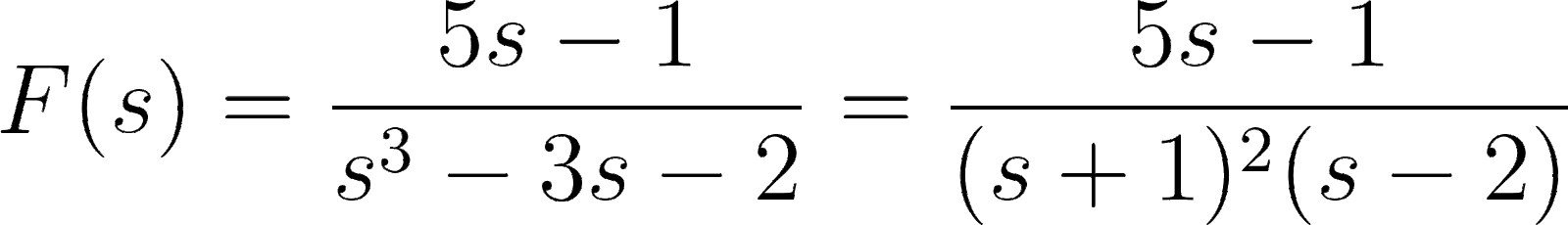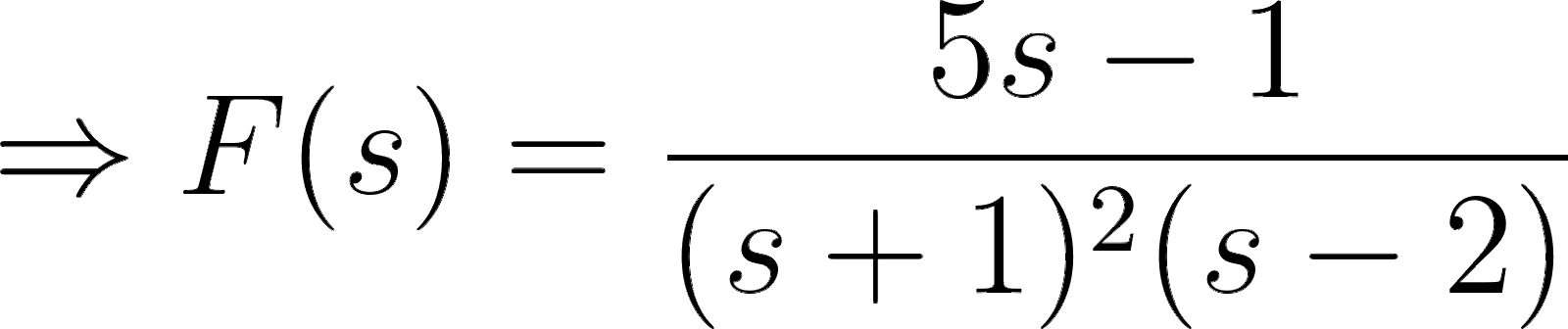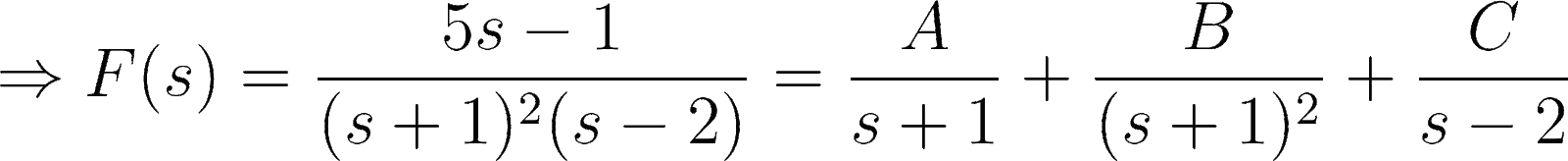Introduction To Electric Circuits - 9 Edition - Chapter 14.4 - Problem P14.4-3
Register Now

Join StudySoup

Get Full Access to Introduction To Electric Circuits - 9 Edition - Chapter 14.4 - Problem P14.4-39781118477502

# Find f(t) when F s 5s 1 s3 3s 2

Introduction to Electric Circuits | 9th Edition

Problem P14.4-3

Find f(t) when F s 5s 1 s3 3s 2

Accepted Solution
Step-by-Step Solution:

Step 1 of 3

GivenPartial fraction expansion has to be used to find the expression for###### Chapter 14.4, Problem P14.4-3 is Solved

Step 2 of 3

Step 3 of 3

Unlock Textbook Solution